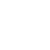Classes (extension) |

# RosslerL : MultiOutUGen : UGen : AbstractFunction : ObjectExtension

Rossler chaotic generator

## Description

A strange attractor discovered by Otto Rossler based on work in chemical kinetics. The system is composed of three ordinary differential equations:

x' = - y - z y' = x + ay z' = b + z(x - c)

The time step amounthdetermines the rate at which the ODE is evaluated. Higher values will increase the rate, but cause more instability. A safe choice is the default amount of 0.05.

## Class Methods

### RosslerL.ar(freq: 22050, a: 0.2, b: 0.2, c: 5.7, h: 0.05, xi: 0.1, yi: 0, zi: 0, mul: 1.0, add: 0.0)

#### Arguments:

 in freq iteration frequency in Hertz a, b, c equation variables h integration time step xi initial value of x yi initial value of y zi initial value of z

## Examples

These first examples treat RosslerL as a single-output UGen (i.e. using x only):

An example utilising the three different outputs as pitch, PWM and pan values (respectively):